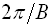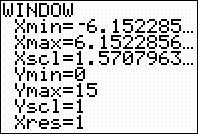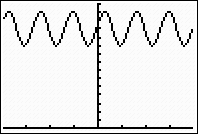Graphing Sinusoidal Functions

 Graph  y = 2sin(3(x - 2)) + 12

This is a sinusoidal equation of the form y = Asin(B(x - C)) + D, where A is the amplitude, B is the frequency, C is the horizontal shift and D is the vertical shift.   The period of this graph will beWhen graphing such equations, it may be necessary to adjust the window to "see" the graph.  If ZOOM #7 Trig is used with this graph, the screen will appear empty.  The vertical shift tells you that the graph has been "raised" by 12 units.  Adjust the yMax and yMin accordingly.  The yMin has been set to 0 for this example to establish a reference point when viewing the graph.

Enter the function.                          Set the MODE to Radian.                   Adjust the ZOOM #7 ZTrigGraph